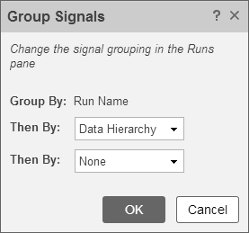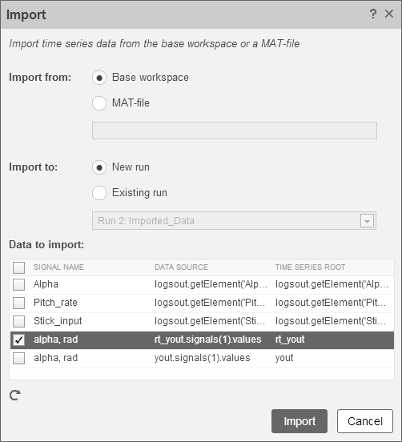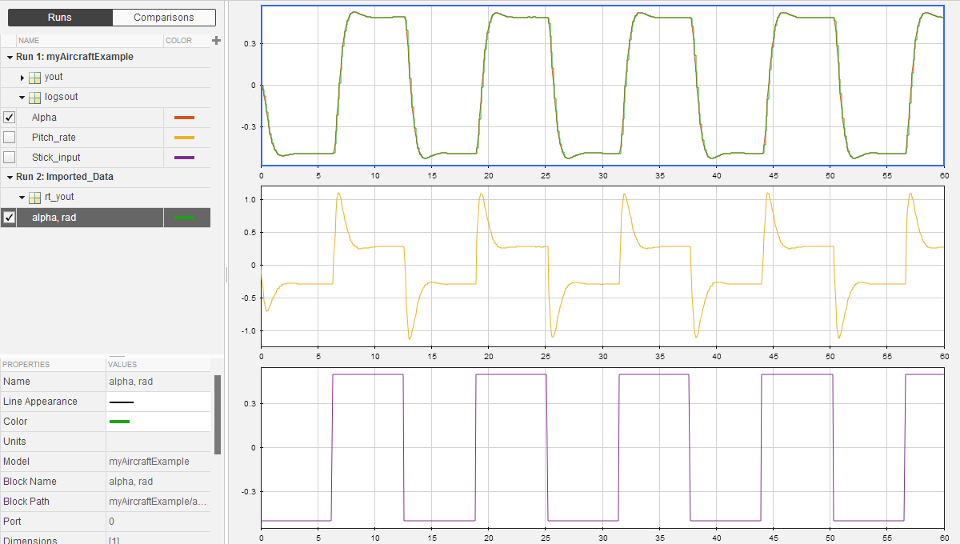## Simulation and Code Comparison

This example shows how to verify the answers computed by code generated from the `slexAircraftExample` model. It shows how to capture and compare two sets of output data. Simulating the model produces one set of output data. Executing the generated code produces a second set of output data.

### Note

To obtain a valid comparison between model output and the generated code, use the same Solver selection and Step size for the simulation run and the build process.

### Configure Signal Data for Logging

Configure the model for logging and recording signal data.

1. Make sure that `slexAircraftExample` is closed. Clear the base workspace to eliminate the results of previous simulation runs. In the Command Window, type:

`clear`

The clear operation clears variables created during previous simulations and all workspace variables, some of which are standard variables that the `slexAircraftExample` model requires.

2. In the Command Window, enter `slexAircraftExample` to open the model.

3. In the model window, choose File > Save As, navigate to the working folder, and save a copy of the `slexAircraftExample` model as `myAircraftExample`.

4. Set up your model to log signal data for signals: `Stick`, `alpha,rad`, and `q, rad/sec`. For each signal:

1. Right-click the signal. From the context menu, select Properties.

2. In the Signal Properties dialog box, select Log signal data.

3. In the Logging name section, from the drop-down list, select `Custom`.

4. In the text field, enter the logging name for the corresponding signal.

Signal NameLogging Name
`Stick``Stick_input`
`alpha,rad``Alpha`
`q, rad/sec``Pitch_rate`

5. Click and .

5. In the Configuration Parameters dialog box:

1. Set Type to `Fixed-step`.

2. Set Format to ```Structure with time```.

3. Clear the States check box.

4. Select the Signal logging check box.

5. Select the Record logged workspace data in Simulation Data Inspector check box.

6. Save the model.

Proceed to Log Simulation Data.

### Log Simulation Data

Run the simulation, log the signal data, and view the data in the Simulation Data Inspector.

1. Run the model. When the simulation is done, on the Simulink® Editor toolbar, the button is highlighted to indicate that new simulation output is available in the Simulation Data Inspector.2. Click the button to open the Simulation Data Inspector.

3. Group the signals:

1. On the Visualize tab, click .

2. In the Group Signals dialog box, select ```Data Hierarchy``` from the Then By list.3. Click OK.

4. Click the `logsout` expander to view the logged signals.

5. Click the Format tab.

6. Click the button and select `3x1` to show three subplots.

7. For each signal:

1. Click the top subplot. A blue border indicates the plot selection.

2. Select the check box next to the `Alpha` signal name. The signal data appears in the subplot.

3. Plot the `Pitch_rate` signal in the middle subplot.

4. Plot the `Stick_input` signal in the bottom subplot.Proceed to Run Executable and Load Data.

### Run Executable and Load Data

You must rebuild and run the `myAircraftExample` executable to obtain a valid data file because you have modified the model.

1. In the Configuration Parameters dialog box, set the MAT-file variable name modifier parameter to `rt_`. `rt_` is prefixed to each variable that you selected for logging in the first part of this example.

2. Click and .

3. Save the model.

4. To generate code, on the C Code tab, click button.

5. When the build is finished, run the standalone program from the Command Window.

`!myAircraftExample`

The executing program writes the following messages to the Command Window.

```** starting the model ** ** created myAircraftExample.mat ** ```
6. Load the data file `myAircraftExample.mat`.

```load myAircraftExample ```

### Tip

For UNIX® platforms, run the executable program in the Command Window with the syntax `!./``executable_name`. If preferred, run the executable program from an OS shell with the syntax `./``executable_name`. For more information, see Run External Commands, Scripts, and Programs (MATLAB).

Proceed to Visualize and Compare Results.

### Visualize and Compare Results

When you follow the example sequence that began in Configure Signal Data for Logging, you obtain data from a Simulink run of the model and from a run of the program generated from the model.

1. To view the execution output for `alpha,rad`, import the data into the Simulation Data Inspector.

1. On the Simulation Data Inspector Visualize tab, click the button to open the Import dialog.

2. Specify Import from as Base workspace.

3. Specify Import to as New run.

4. To the left of Signal Name, click the check mark to clear the check boxes.

5. Select the check box for the `alpha,rad` data where the Time Series Root is `rt_yout`.

6. Click .The selected data is now under Run 2: Imported_Data.

2. View a plot of the executed data.

1. Click the `rt_yout` expander.

2. Click the top subplot and select the check box next to the `alpha, rad` signal name. The signal data appears in the top subplot.

The `alpha, rad` signal from Run 1 and Run 2 overlap in the subplot because the signals are equivalent.It is possible to see a very small difference between simulation and code generation results. A slight difference can be caused by many factors, including:

• Different compiler optimizations

• Statement ordering

• Run-time libraries

For example, a function call such as `sin(2.0)` can return a slightly different value depending on which C library you use. Such variations can also cause differences between your results and these results.

### Compare States for Simulation and Code Generation

The order in which Simulink logs states during simulation is different than the order in which Simulink Coder™ logs states during code generation. If you want to compare states between simulation and code generation, sort the states by block name.

For example, by default, Simulink exports state data to the MATLAB variable, `xout`. Simulink Coder exports state data to the variable `rt_xout`. To sort the state data for these variables, enter the following commands in the MATLAB Command Window:

```[~,idx1]=sort({xout.signals.blockName}); xout_sorted=[xout.signals(idx1).values]; [~,idx2]=sort({rt_xout.signals.blockName}); rt_xout_sorted=[rt_xout.signals(idx2).values];```

You can confirm that the logging order is the same between code generation and simulation by entering the following command in the MATLAB Command Window:

`isequal(xout_sorted, rt_xout_sorted)`